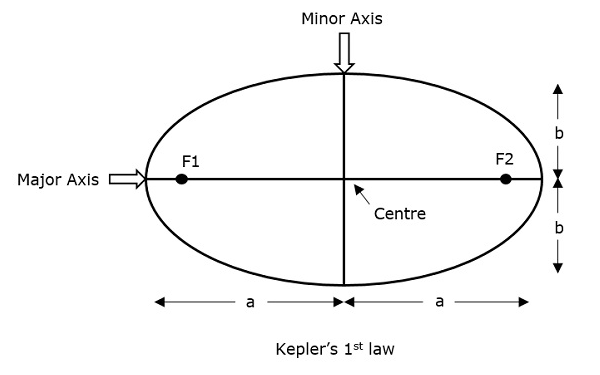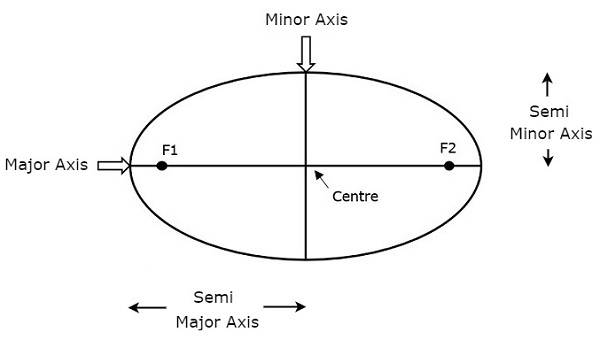# satellite communication viva questions as per GTU syllabus

Q-1. Explain Kepler”s Law.

Ans:-

Kepler’s First Law

Kepler’s first law states that the path followed by a satellite around its primary (the earth) will be an ellipse. This ellipse has two focal points (foci) F1 and F2 as shown in the figure below. The Center of mass of the earth will always present at one of the two foci of the ellipse. This ellipse has two focal points (foci) F1 and F2 as shown in the figure below. The Center of mass of the earth will always present at one of the two foci of the ellipse.If the distance from the center of the object to a point on its elliptical path is considered, then the farthest point of an ellipse from the center is called apogee and the shortest point of an ellipse from the center is called as perigee.

Eccentricity “e” of this system can be written as −

e=a2-b2

a

Where, a & b are the lengths of semi major axis and semi minor axis of the ellipse respectively.

For an elliptical path, the value of eccentricity (e) always lies between 0 and 1, i.e. 00 < ee < 11, since a is greater than b. Suppose, if the value of eccentricity (e) is zero, then the path will be no more in an elliptical shape, rather it will be converted into a circular shape.

## Kepler’s Second Law

Kepler’s second law states that for equal intervals of time, the area covered by the satellite will be the same with respect to the center of mass of the earth. This can be understood by taking a look at the following figure.

Assume, the satellite covers p1 and p2 distances in the same time interval. Then, the areas B1 and B2 covered by the satellite at those two instances are equal.

## Kepler’s Third Law

Kepler’s third law states that the square of the periodic time of an elliptical orbit is proportional to the cube of its semi-major axis length.

Q-2. What is the Carrier to noise ratio (CNR)?

Ans:- Carrier to noise ratio, also known as CNR and C/N is a signal-to-noise ratio of a modulated signal. In simple terms, it is a measure of the received carrier strength in relation to the strength of the noise received (Margaret Rouse, Search Networking, CARRIER-TO-NOISE RATIO (CNR OR C/N), n.d.)

Q-3.  What is the Earth eclipse of Satellite?

Ans:-  A satellite is said to be in eclipse when the earth or moon prevents sunlight from reaching it. If the earth’s equatorial plane coincides with the plane of the earth’s orbit around the sun, the geostationary orbit will be eclipsed by the earth. This is called the earth eclipse of the satellite.

Q-4. Explain Orbital Elements in detail?

Ans:-

Orbital elements are the parameters, which are helpful for describing the orbital motion of satellites. Following are the orbital elements.

• Semi-major axis
• Eccentricity
• Mean anomaly
• Argument of perigee
• Inclination
• The right ascension of ascending node

The above six orbital elements define the orbit of earth satellites. Therefore, it is easy to discriminate one satellite from other satellites based on the values of orbital elements.

### Semi-major axis

The length of the Semi-major axis (a) defines the size of the satellite’s orbit. It is half of the major axis. This runs from the center through a focus to the edge of the ellipse. So, it is the radius of an orbit at the orbit’s two most distant points.Both the semi-major axis and semi-minor axis are represented in the above figure. Length of semi-major axis (a) not only determines the size of the satellite’s orbit but also the time period of revolution.

If the circular orbit is considered as a special case, then the length of the semi-major axis will be equal to the radius of that circular orbit.

### Eccentricity

The value of Eccentricity (e) fixes the shape of the satellite’s orbit. This parameter indicates the deviation of the orbit’s shape from a perfect circle.

If the lengths of the semi-major axis and semi-minor axis of an elliptical orbit are a & b, then the mathematical expression for eccentricity (e) will be

e=a2b2−−−−−−√ae=a2−b2a

The value of eccentricity of a circular orbit is zero since both a & b are equal. Whereas, the value of eccentricity of an elliptical orbit lies between zero and one.

### Mean Anomaly

For a satellite, the point which is closest to the Earth is known as Perigee. Mean anomaly (M) gives the average value of the angular position of the satellite with reference to perigee.

If the orbit is circular, then the Mean anomaly gives the angular position of the satellite in the orbit. But, if the orbit is elliptical, then the calculation of the exact position is very difficult. At that time, Mean anomaly is used as an intermediate step.

### Argument of Perigee

Satellite orbit cuts the equatorial plane at two points. The first point is called a descending node, where the satellite passes from the northern hemisphere to the southern hemisphere. The second point is called as ascending node, where the satellite passes from the southern hemisphere to the northern hemisphere.

The argument of perigee (ω) is the angle between ascending node and perigee. If both perigee and ascending node are existing at the same point, then the argument of perigee will be zero degrees

The argument of perigee is measured in the orbital plane at earth’s center in the direction of satellite motion.

### Inclination

The angle between the orbital plane and the earth’s equatorial plane is known as an inclination (i). It is measured at the ascending node with the direction being east to north. So, inclination defines the orientation of the orbit by considering the equator of the earth as a reference.

### Right Ascension of Ascending node

We know that ascending node is the point, where the satellite crosses the equatorial plane while going from the southern hemisphere to the northern hemisphere.

Right Ascension of ascending node (Ω) is the angle between the line of Aries and ascending node towards the east direction in the equatorial plane. Aries is also called vernal and equinox.

The satellite’s ground track is the path on the surface of the Earth, which lies exactly below its orbit. The ground track of a satellite can take a number of different forms depending on the values of the orbital elements.

Q-5. Full form of  FSS?

Ans:- Fixed-Satellite Services.

Q-6. What is IPBO?

Ans:-  Input Back-Off (IPBO) is the power level at the input of RF amplifier relative to input power which produces maximum output power.

Q-7. What is OPBO?

Ans:- Both the Output backoff(OPBO) and Input Backoff(IPBO) are used to determine the operating power levels required in a satellite transponder TWTA or RF Power Amplifier to function in linear region

Q-8.  What is the Full Form of (EIRP)?

Q-9. What is descending node?

Ans:-  The descending node is the point at which an orbiting body passes from the northern side of a reference plane to the southern side, hence ‘descending’.

Q-10. What is ascending node?

Ans:- The ascending node is the point where the orbit of the object passes through the plane of reference, as seen in the adjacent image.

Q-11. What is a line of nodes?

Ans;- The line of nodes is a line that joins the ascending node and the descending node of an orbit.

Q-12. Define the Apogee.

Ans:- Apogee is the point farthest from the earth. Perigee is the point of the closest approach to earth.

Q-13. Define Argument of Perigee.

Ans:- The Argument of Perigee (w) is defined as the angle within the satellite orbit plane that is measured from the Ascending Node (W) to the perigee point (p) along the satellite’s direction of travel.

Q-14. What is  Orbital inclination?

Ans;- orbital inclination measures the tilt of an object’s orbit around a celestial body. It is expressed as the angle between a reference plane and the orbital plane or axis of direction of the orbiting object.

Q-15. What is the speed of GEO satellites?

Ans:-Geostationary orbits (GEO) are used for tv, and communication satellites. … To maintain an orbit that is 22,223 miles (35,786 km) above Earth, the satellite must orbit at a speed of about 7,000 mph (11,300 kph).

### 1 thought on “Satellite communication viva questions”

1.This weeb site really has alll off the informatjon I needed
aboiut this subject and didn’t know who too ask.